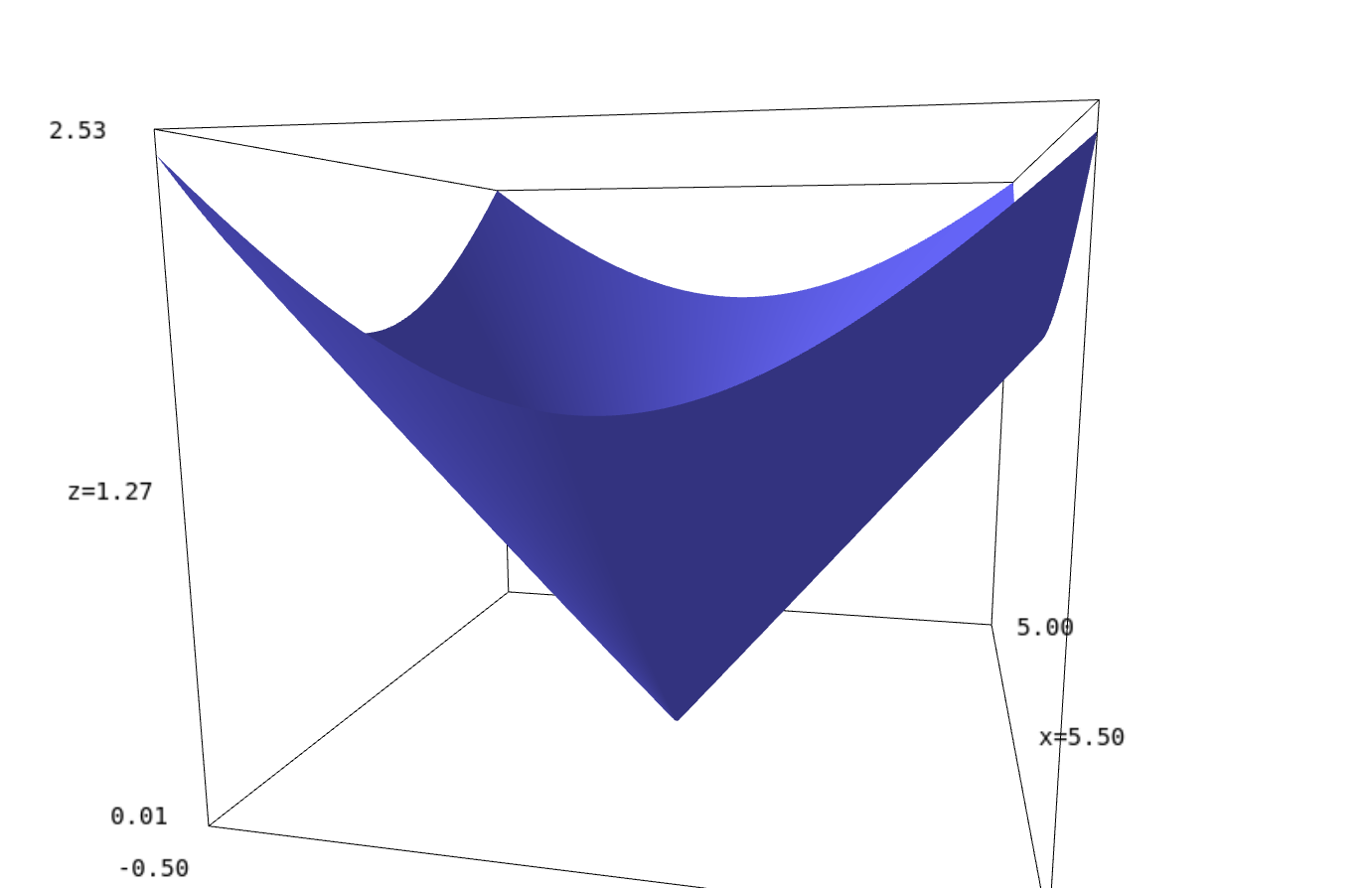# Cannot evaluate symbolic expression to a numerical value

I'm trying to do this:  (sqrt(10*y*(10-y))+sqrt(1000)*acos(sqrt(y/10))-15*sqrt(2*6.673*10^(-11)*50000000000)).roots( ring=RealField(100))  Unfortunately I get the error in the title. Also any other way of solving the above equation numerically would be appreciated. I was able to do it in maxima using find_root but was hoping for a better function (one that doesn't require specifying an interval). I couldn't use find_root in sage because it returns the error 'unable to simplify to float approximation' and ofcourse solve doesn't return explicit solutions.

edit retag close merge delete

Sort by » oldest newest most votedFrom the documentation of roots:

Return roots of self that can be found exactly, possibly with multiplicities. Not all roots are guaranteed to be found.

Warning: This is not a numerical solver

and

ring - a ring (default None): if not None, convert self to a polynomial over ring and find roots over ring

The latter explains why you get the error in the title: the expression is not a polynomial, so the conversion fails.

The expression itself is only defined as a real number if y is between 0 and 10 (due to the $\sqrt{y(10-y)}$), so:

sage: var('y')
sage: f = sqrt(10*y*(10-y))+sqrt(1000)*acos(sqrt(y/10))-15*sqrt(2*6.673*10^(-11)*50000000000)
sage: f.find_root(0,10)
5.5672155196677675
sage: find_root(f,0,10)
5.5672155196677675


By plotting f you see that it's the only root.

In general there is no magic method to find an interval where a root may live. It is however always a good idea to check the domain of definition first.

more

1

A visual exploration of the complex region around 0 suggests that the real root approximated by rburing is the only one "close to 0" :

complex_plot(lambda u:(sqrt(10*u*(10-u))+sqrt(1000)*acos(sqrt(u/10))-15*sqrt(2*6.673*10^(-11)*50000000000)),(-10,10),(-10,10))A 3D plot of the modulus closer to the real root suggests that this root is indeed unique :Further analytical work (finding majorants/minorants of moduli) may prove the absence of roots outside this region and the uniqueness of the real root.

This is left to the reader as an exercise ;-)...